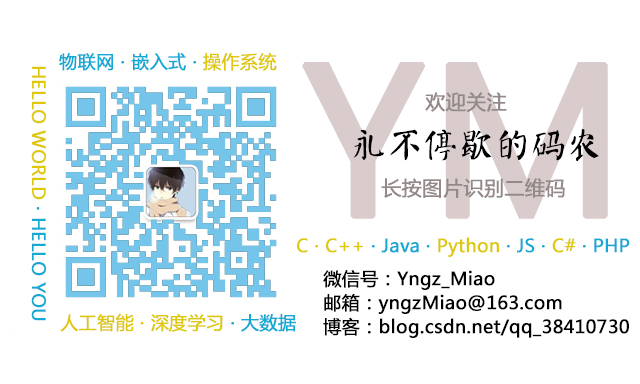# 【C】C语言指针（包括：指针与数组、指针与字符串、指针与函数）

## 指针概述

### 指针的概念

• 直接访问：通过变量来实现，因为变量是内存中某一块存储区域的名称；
• 间接访问：通过指针来实现。指针并不是用来存储数据的，而是用来存储数据在内存中的地址的。

### 指针的类型

• 指针的类型：将指针声明语句中去掉指针名字，而剩下的部分；
• 指针所指向的类型：将指针声明语句中去掉指针名字和指针声明符（*），而剩下的部分。例如：
int* ptr;

### 运算符&和*

C语言中提供了地址运算符（&）来表示变量的地址，指针运算符（*）来表示获取指针变量所指向的变量的值。

&*p和*&p的区别：

• &*p：相当于&(*p)，首先进行一次*p运算再取地址。若p是一个非指针变量，*p是非法的；若p是一个指针变量，&(*p)=p；
• *&p：相当于*(&p)，先取地址再做*运算。若p是一个非指针变量，*(&p)=p；若p是一个指针变量，*(&p)=p。

p为指针变量时，表示指针的值为一个地址，但是这个地址也存储在一个变量里，也存储在一个内存单元里。&p的意义，就是存放了一个地址的内存单元的地址。

#include<stdio.h>

int main()
{
int a=0;
int* p = &a;

printf("%d %d\n", a, &a);
printf("%d %d %d\n", p, *p, &p);
printf("%d %d\n", a, *&a);
printf("%d %d %d\n", p, *&p, &*p);

return 0;
}

0 5240964
5240964 0 5240952
0 0
5240964 5240964 5240964

## 指向变量的指针

### 指针变量的使用

int* p = 2;            /* 错误的赋值，需要指向一个地址或0 */
int* p = 0;            /* 正确的赋值，表示指针指向空 */

### 指针变量作为函数函数

#include<stdio.h>

void swap(int* point1, int* point2);

int main()
{
int a,b;
int* p1 = &a;
int* p2 = &b;

scanf_s("%d %d", &a, &b);
swap(p1, p2);
printf("%d %d\n", a, b);
printf("%d %d\n", *p1, *p2);

return 0;
}

void swap(int* point1, int* point2) {
int temp;
temp = *point1;
*point1 = *point2;
*point2 = temp;
}

1 2
2 1
2 1

#include<stdio.h>

void swap(int point1, int point2);

int main()
{
int a,b;

scanf_s("%d %d", &a, &b);
swap(a, b);
printf("%d %d\n", a, b);

return 0;
}

void swap(int point1, int point2) {
int temp;
temp = point1;
point1 = point2;
point2 = temp;
}

1 2
1 2

## 指针与一维数组

### 指针与一维数组的关系

int a, *p;
p = a;                /* 数组名表示数组的首地址，可将数组名直接赋值给指针变量 */
p = &a;            /* 数组第一个元素的地址也就是整个数组的首地址 */

p=a，并不是将a数组的所有元素都赋值给p，只是把数组a的第一个元素的地址即首地址赋值给指针p。C语言规定：数组名代表数组的首地址，也就是第0个元素的地址。

#include <stdio.h>

int main()
{
int a = { 0,1,2,3,4,5,6,7,8,9 };
int* p;
p = &a;

printf("%d %d %d %d\n", a, &a, &a, a);
printf("%d %d\n", p, *p);

return 0;
}

0 2948652 2948652 2948652
2948652 0

### 指针的运算

• 数组指针变量向前向后移动一个位置和地址加1或减1在概念上是不同的。因为数组的元素可以由不同的数据类型，所占用的字节长度也是不同的。如指针变量加1，即表示指针变量指向下一个元素的首地址，而不是在原地址的基础上加1；
• 指针变量的加减运算只能对数组指针变量进行，对只想其他数据类型的指针变量做加减运算是毫无意义的。
• 两个指针变量之间的运算：只有指向同一数组的两个指针变量才能进行运算，否则运算是毫无意义的。
• 两指针变量相减：两指针变量相减所得之差，是两个指针所指向的数据元素之间相差的元素个数，乘以该数组每个数据元素的长度（字节数）；
• 两指针变量相加：两指针变量不能进行加法运算，毫无实际意义。

## 指针与二维数组

### 二维数组与一维数组的关系

int a[M][N];

#include <stdio.h>

int main()
{
int a = { 1,2,3,4,5,6 };
int i;
int* p;
p = *a;

for (i = 0; i < 6; i++) {
printf("%d\n", *p);
p++;
}

return 0;
}

1
2
3
4
5
6

	int* p;
p = *a;

### 数组指针（行指针）

存储类型 数据类型(*指针变量名)[元素个数];
int (*p);

• *p两端的括号一定不能漏掉，如果写成*p的形式，由于[]的运算级别高，所以p先与[]结合，是数组，然后在与前面的*结合，*p是指针数组；
• p是一个行指针，它只能指向一个包含n个元素的一维数组，不能指向一位数组的元素；
• 可以理解为int *p，即p指向的是一个int类型的一维数组。

#include<stdio.h>

int main()
{
int array = { 1,2,3,4,5,6 };
int i, j;
int(*p);
p = array;
for (i = 0; i < 2; i++) {
for (j = 0; j < 3; j++) {
printf("%d %d %d\n", p[i][j], *(*(p+i)+j), *(p[i]+j));
}
}

return 0;
}

1 1 1
2 2 2
3 3 3
4 4 4
5 5 5
6 6 6

• 它是一个指针，所以(*p)按照优先级来算，p与*先结合，指针；
• 由于是行指针，数组指针p可以通过p+1来转换到下一行，那么列中怎么操作呢？通过[]内的数字来访问；或者对行指针取*运算，可以变成列指针。

int a = {1, 2, 3, 4};
int (*p);
p = a;

• a是一个二维数组名，类型是指向一维数组的指针（行指针），不是变量，a的值是指针常量，即不能有a++或者a=p这些操作。a指向这块连续空间的首地址，值是&a；
• a是一维数组名，类型是指向整型的指针（列指针），值是&a，这个值是一个常量；
• a是一维数组名，类型是指向整型的指针（列指针），值是&a，这个值是一个常量；
• p是一个数组指针变量，指向一维数组的指针变量，值是&a。可以执行p++;p=a等操作；
• a+1表示指向下一行元素，也可以理解为指向下一个一维数组；
• *(a+1)是取出第一行的首地址（列指针）；
• a+1是指向第0行第1个元素，也可以理解为指向一维数组a的第一个元素；
• p+1同a+1；
• *(p+1)同*(a+1)；

 表示方法 意义 a、a、*(a+0)、*a、&a 0行0列元素的地址 a+i、*(a+i)、&a[i]、&a[i]、a[i] i行0列元素的地址 *(a[i]+j)、*(*(a+i)+j)、a[i][j] i行j列元素的地址

#include <stdio.h>

int main()
{
int a = { 1,2,3,4,5,6 };
int (*p);
p = a;

printf("%d\n%d\n", sizeof a, sizeof p);

return 0;
}

24
4

### 指针数组

存储类型 数据类型 *指针变量名[元素个数];
int* p;

#include<stdio.h>

int main()
{
int a = { 1,2,3,4,5,6 };
int i, j;
int* p = { a, a };

for (i = 0; i < 2; i++) {
for (j = 0; j < 3; j++) {
printf("%d\n", *(p[i] + j));
}
}

return 0;
}

1
2
3
4
5
6

int* p;                /* 二维数组有两行 */
int (*p);                /* 二维数组有两列 */

char* p = { "Hello", "World" };

## 指针与字符串

char* p;
p = "Hello World!";

char* p;
p = "Hello World!";
printf("%c\n", *p);            /* H */
printf("%s\n", p);            /* Hello World! */

• 存储内容不同：字符串指针变量本身是一个变量，存储的是字符串的首地址。而字符串本身存放在以该首地址为首的一块连续的内存空间中并以'\0'作为串的结束。字符数组中存储的是字符串本身；
• 赋值方式不同：对字符串指针变量的赋值方式，可以使用如下两种：
char* c="Hello World!";            /* 正确 */
char* c;
c="Hello World!"            /* 正确 */

char c = "Hello World!";            /* 正确 */
char c;
c = "Hello World!"            /* 错误 */
• 存储器空间分配不同：字符串指针变量只会给这个变量分配空间，而字符串数组会给整个数组分配空间。这部分介绍的归根结底仍然是字符数组和字符串变量之间的本质区别，先看两个例子：

#include<stdio.h>

int main()
{
char* p;
scanf_s("%s", p, 10);

return 0;
}

#include<stdio.h>

int main()
{
char p;
scanf_s("%s", p, 10);

return 0;
}

## 指向指针的指针

存储类型 数据类型 **指针变量名;

#include <stdio.h>

int main()
{
char* p = { "Hello","World","How","Are","You" };
char** pp;
pp = p;
int i;
for (i = 0; i < 5; i++) {
printf("%s %s\n", *pp, p[i]);
pp++;
}

return 0;
}

Hello Hello
World World
How How
Are Are
You You

p是一个指针数组，数组的元素都是指针。p是数组名，数组名表示着数组的首地址，也就是数组首元素的地址，而数组首元素是一个指针。归根结底，数组名也就是指向指针的指针。所以pp=p，是没有问题的。

int a = { 1,2,3,4,5,6 };
int** p;
p = a;                /* 错误 */

## 指针与函数

### 函数指针

数据类型 (*函数指针名)(形参列表);
int (*f)(int,int);

指针变量名 = 函数名;
f = fun;                /* 函数指针的赋值 */
(*f)(a,b);                /* 等价于调用函数fun(a,b); */

#include <stdio.h>

int max(int x, int y);

int main()
{
int(*p)(int, int);
p = max;
int i;
i = (*p)(2, 3);
printf("%d\n", i);

return 0;
}

int max(int x, int y) {
int z;
if (x > y)
z = x;
else
z = y;
return z;

}

### 指针函数

类型名 *函数名(参数表列);

#include <stdio.h>

int* max(int a[], int* n);

int main()
{
int a = { 1,2,3,4,5 };
int sum;
int* value;
value = max(a, &sum);
printf("%d %d\n", *value, sum);

return 0;
}

int* max(int a[], int* n) {
int i;
int* tem1 = &a;
*n = a;
for (i = 1; i < 5; i++) {
if (a[i] > *tem1)
*tem1 = a[i];
*n += a[i];
}
return tem1;
}

5 15

## void指针类型

C语言中void指针的定义格式为：

void* p;

int* p1;
void* p2;
p2 = p1;
p1 = (int*) p2;

void关键字在C语言中的注意事项：

• 如果函数没有返回值，用声明为void类型。在C语言中，凡不加返回值类型限定的函数，就会被编译器默认返回整数型处理，包括main函数；
• 如果函数不接受参数，应指明参数为void。在C语言中，可以给五参数的函数传送任意类型的参数，但若函数要不接受参数，应指明其参数为void；
• 如果函数的参数为任意类型指针，应声明其参数为void*，即void指针型。void不能代表一个真实的变量，在C语言中定义一个void类型的变量是不合法的；
• 不能对void指针进行算术运算，也就是说++，--运算都是不合法的；
• void指针不等同于空指针，void指针是没有指向任何数据类型的指针，即其指向的是一块空白的内存区域；而空指针是指向NULL的指针，指向的是一个具体的区域。09-2812万+02-033万+
04-213752
03-134693
07-263279
12-034839
01-132025
10-262573
06-301万+
©️2020 CSDN 皮肤主题: 程序猿惹谁了 设计师:白松林